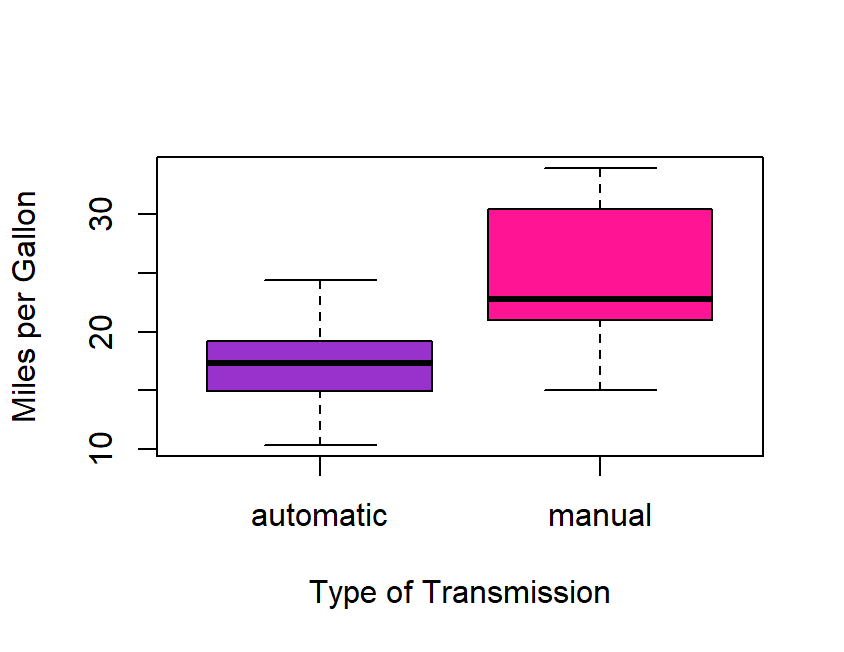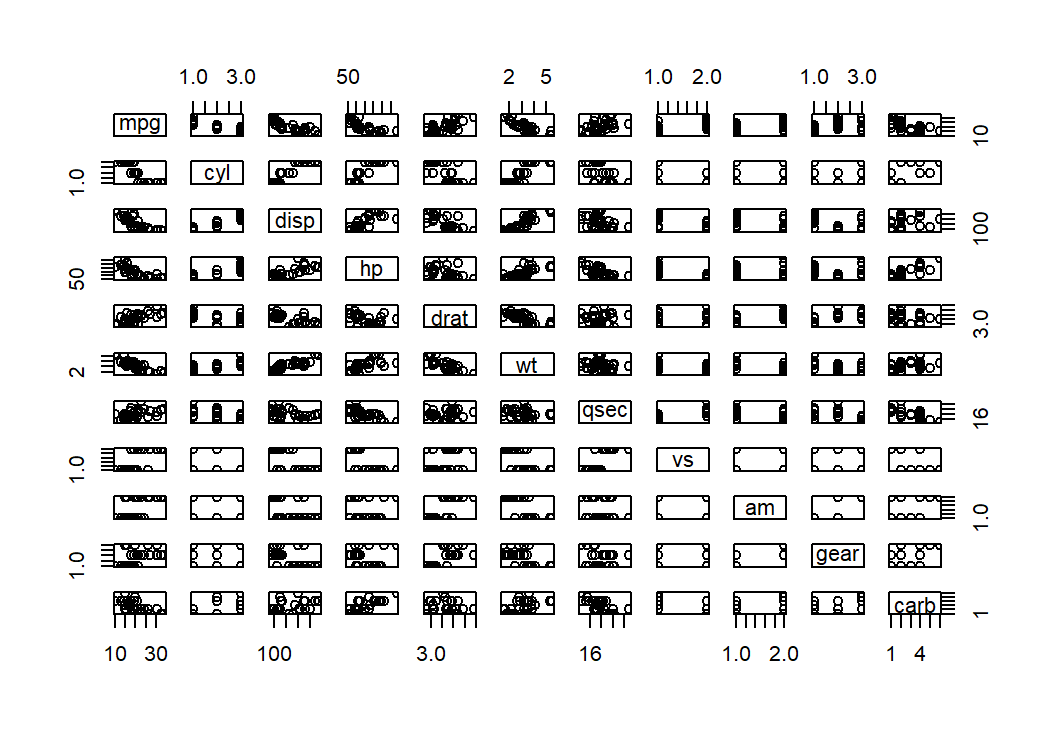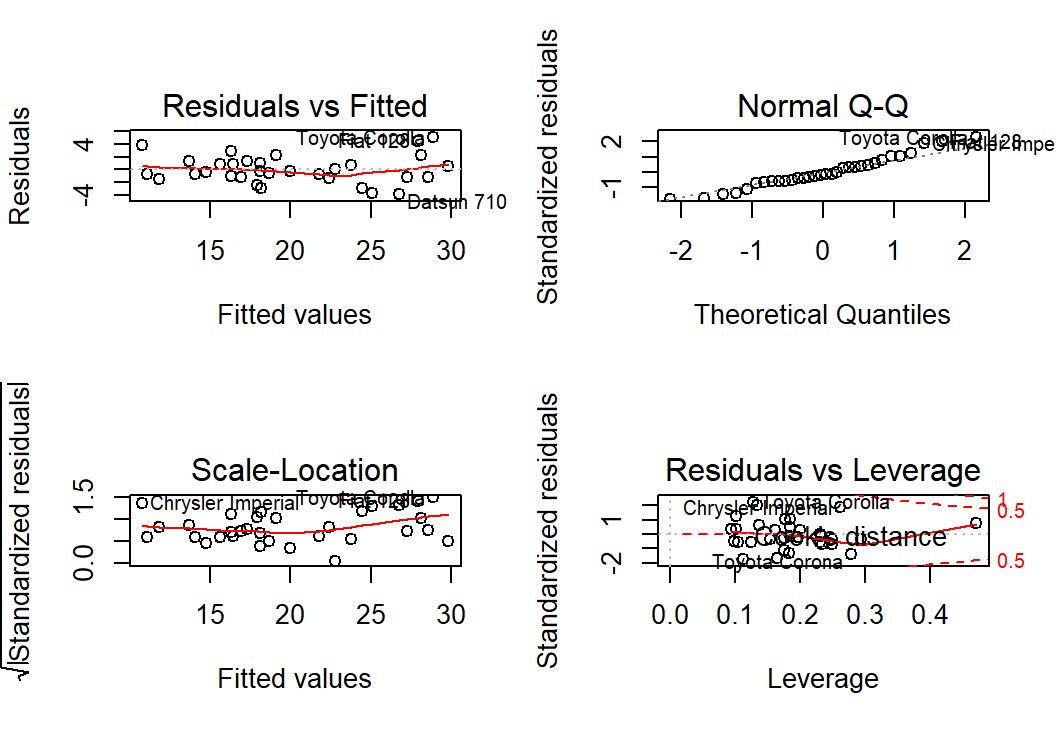## Executive Summary

This is a project for the Regression Models course on Data Science by Johns Hopkins University via Coursera. In this report we look into a collection of cars and explored on the relationship between a set of variables and miles per gallon as the the outcome. Inferential analysis show that manual (24.39) is better compared to automatic (17.15) in terms of mpg. However, results of the regression analysis using the best model show that when significant predictors such as cylinder(cyl), horsepower(hp) and weight(wt) are included in the model, the type of transmission seems to be insignificant (p > 0.05) eventhough shifting from auto to manual increases mpg by 1.81.

## Exploratory Data Analysis

In this project let us first open the “datasets”" library and load the mtcars dataset.

#Load the mtcars dataset
library(datasets)
data <- mtcars
#Let us check the variables included in the loaded data and its structure
str(data) #Convert numeric variables: cyl, vs, gear, carb and am into factor
data$cyl <- factor(data$cyl); data$vs <- factor(data$vs); data$gear <- factor(data$gear)
data$carb <- factor(data$carb); data$am <- factor(data$am)
#Since Transmission is our main independent variable, let's label it accordingly
data$am <- factor(data$am, levels = c("0", "1"), labels = c("automatic", "manual"))
#Conduct a test for normality to find out whether the dependent variable assumes a normal distribution
library(nortest)
#The Anderson-Darling test for normality is used, a p-value of >0.05 indicates that the mpg data
#assumes normal distribution thus we can use the mean as the measure for central tendency.
ad.test(data$mpg)$p
##  0.1207371

To show the mpg means of automatic versus manual, a boxplot is made which is presented in Appendix 1.

## Inferential Analysis (difference in mpg between automatic and manual)

Inferential analysis is done to determine whether observation of the sample, manual transmission having more mpg compared to automatic, reflects that of the population with a 95% level of confidence. The null hypothesis that there is no significant difference in mpg between automatic and manual is considered.

t.test(data$mpg~data$am)
##
##  Welch Two Sample t-test
##
## data:  data$mpg by data$am
## t = -3.7671, df = 18.332, p-value = 0.001374
## alternative hypothesis: true difference in means is not equal to 0
## 95 percent confidence interval:
##  -11.280194  -3.209684
## sample estimates:
## mean in group automatic    mean in group manual
##                17.14737                24.39231

A p-value of < 0.05 and a confidence interval which does not include zero (-11.28 to -3.21) indicates that the null hypothesis is to be rejected thus the mpg between automatic and manual transmission has significant difference. As such, the said observation of the sample may also hold true for the population wherein the manual transmission seem to have more miles travelled per gallon as compared to the automatic transmission.

## Regression Analysis

A regression analysis is conducted to determine the unit change in the dependent variable given the independent variable. Regression analysis where mpg is the outcome and transmission type is the predictor is done first to be informed of the variance it explains via the R-squared.

fit1 <- lm(mpg~am, data = data)
summary(fit1)
##
## Call:
## lm(formula = mpg ~ am, data = data)
##
## Residuals:
##     Min      1Q  Median      3Q     Max
## -9.3923 -3.0923 -0.2974  3.2439  9.5077
##
## Coefficients:
##             Estimate Std. Error t value Pr(>|t|)
## (Intercept)   17.147      1.125  15.247 1.13e-15 ***
## ammanual       7.245      1.764   4.106 0.000285 ***
## ---
## Signif. codes:  0 '***' 0.001 '**' 0.01 '*' 0.05 '.' 0.1 ' ' 1
##
## Residual standard error: 4.902 on 30 degrees of freedom
## Multiple R-squared:  0.3598, Adjusted R-squared:  0.3385
## F-statistic: 16.86 on 1 and 30 DF,  p-value: 0.000285

The adjusted r-squared is only 34%. It is the variance in mpg accounted by the type of transmission which is somewhat low. In search of the best model, a scatter plot matrix has been generated to see associations of variables with mpg; this is shown in Appendix 2. A stepwise regression analysis is also done having both backward and forward directions considered. Given a lengthy output (which, if presented, will make this report exceed 5 pages), only the summary of the best model is presented below. For reproducibility, the code which returns the lengthy output of the stepwise regression which determines the best model is also included.

#Creating model which includes all variables as predictors
fit2 <- lm(mpg~., data=data)
#Determining the best model using stepwise regression analysis
best <- step(fit2, direction = "both")

Multiple models from the stepwise regression were compared using the Aikake Information Criterion (AIC). The model with the lowest AIC thus accounting for more variance in the dependent variable mpg is as follow:

summary(best)
##
## Call:
## lm(formula = mpg ~ cyl + hp + wt + am, data = data)
##
## Residuals:
##     Min      1Q  Median      3Q     Max
## -3.9387 -1.2560 -0.4013  1.1253  5.0513
##
## Coefficients:
##             Estimate Std. Error t value Pr(>|t|)
## (Intercept) 33.70832    2.60489  12.940 7.73e-13 ***
## cyl6        -3.03134    1.40728  -2.154  0.04068 *
## cyl8        -2.16368    2.28425  -0.947  0.35225
## hp          -0.03211    0.01369  -2.345  0.02693 *
## wt          -2.49683    0.88559  -2.819  0.00908 **
## ammanual     1.80921    1.39630   1.296  0.20646
## ---
## Signif. codes:  0 '***' 0.001 '**' 0.01 '*' 0.05 '.' 0.1 ' ' 1
##
## Residual standard error: 2.41 on 26 degrees of freedom
## Multiple R-squared:  0.8659, Adjusted R-squared:  0.8401
## F-statistic: 33.57 on 5 and 26 DF,  p-value: 1.506e-10
fit3 <- lm(mpg~cyl+hp+wt+am, data=data) #this is the best model

As shown above, the best model includes cylinder (cyl), horsepower(hp) and weight(wt) together with transmission type(am) as predictors. This model has the lowest AIC (61.65) and highest adjusted r-squared (84%) compared to other models created during the stepwise regression (as previously mentioned, the code for stepwise regression is included above, however, the results were hidden due to a lengthy output which will make this report exceed 5 pages if presented). As such, a model which includes cyl, hp, wt, and am accounts for 84% of the variance observed in the mpg. However, it can be noted that cyl(4 and 6), hp and wt were the significant predictors leaving out transmission type (p > 0.05; p= 0.21).

### Residuals and Diagnostics

An examination of the residual plots of the best model has been made. As shown in Appendix 3, the residuals vs. fitted plot does not seem to resemble any pattern thus no concerns for heteroscedasticity. The scale-location plot also does not show any pattern or trend among the residuals. The normal q-q plot presents the points almost lined up thus indicating that it assumes a normal distribution. The residuals vs leverage plot show that there are some outliers; particular outliers of interest were identified as Chrysler Imperial and Toyota Corona. Given the interesting leverage points, they are further examined using regression diagnostics via the influence measures in R.

lvrg <- hatvalues(fit3)
tail(sort(lvrg),3) #highest hatvalues
##       Toyota Corona Lincoln Continental       Maserati Bora
##           0.2777872           0.2936819           0.4713671
inflnce <- dfbetas(fit3)
tail(sort(inflnce[,6]),3) #highest dfbetas
## Chrysler Imperial          Fiat 128     Toyota Corona
##         0.3507458         0.4292043         0.7305402

As shown above, the points (Chrysler and Coronoa) that contributed to the change in values when included in the model are consistent with the results of the residuals vs leverage plots.

### Inferential Analysis (difference between fit1 and the best model)

To further confirm previous observations, ANOVA has been conducted between the previous model (fit1) and the best model (fit3). The aforementioned considers a null hypothesis that cyl, hp and wt do not contribute to the accuracy of the model.

anova(fit1, fit3)

Given a p-value of < 0.05, we reject the null hypothesis that cyl, hp and wt do not contribute to the accuracy of the model.As such, it can be noted that fit3 significantly differs with fit1 and that when these variables are added to the model fit3 seem to improve accuracy.

## Appendix

Appendix 1. Boxplot comparing the mpg means of automatic versus manual transmission

boxplot(data$mpg~data$am, col = c("darkorchid", "deeppink"),
ylab = "Miles per Gallon", xlab = "Type of Transmission")Appendix 2. Scatter Plot Matrix

pairs(mpg~., data=data)Appendix 3. Residual Plots

par(mfrow = c(2,2))
plot(fit3)